Dipole Correlation Method for Determination of Magnetization Directionunder the Influence of Remanent Magnetization | OMICS International
Journal of Remote Sensing & GIS

# Dipole Correlation Method for Determination of Magnetization Directionunder the Influence of Remanent Magnetization

 Lei Shi1*, Lianghui Guo2, Shi Chen1, Weimin Xu1 1Institute of Geophysics, China Earthquake Administration, Beijing 100081, China 2Institute of Geology, Chinese Academy of Geological Sciences, Beijing 100037, China Corresponding Author : Lei Shi Institute of Geophysics, China Earthquake Administration Beijing, 100081, China Tel: 861068729401 E-mail: [email protected] Received November 21, 2013; Accepted December 13, 2013; Published December 20, 2013 Citation: Shi L, Guo L, Chen S, Xu W (2013) Dipole Correlation Method for Determination of Magnetization Direction under the Influence of Remanent Magnetization. J Geophys Remote Sensing S4:001. doi:10.4172/2469-4134.S4-001 Copyright: © 2013 Shi L, et al. This is an open-access article distributed under the terms of the Creative Commons Attribution License, which permits unrestricted use, distribution, and reproduction in any medium, provided the original author and source are credited.

Visit for more related articles at Journal of Remote Sensing & GIS

#### Abstract

Processing and interpretation of magnetic data usually require information of total magnetization direction. However, under the effects of remanant magnetization, total magnetization direction is different from induced magnetization direction, which makes data processing and interpretation complexity. In this paper, we present a new method by cross-correlation of magnetic dipole source for determination of magnetization direction from relatively isolated and approximate equiaxial magnetic total field anomaly. This method calculates cross-correlation coefficient between observed magnetic total field anomaly and theoretical magnetic total field anomaly caused by a magnetic dipole source, by using a set of varying parameters of positions and total magnetization direction of dipole source for trial and error. The corresponding magnetization direction of maximum correlation coefficient is regarded as estimated total magnetization direction. Tests on both synthetic data and aeromagnetic data of a mineral exploration in northwestern China indicate this method reliably and effectively estimates the magnetization direction from relatively isolated and approximate equiaxial magnetic total field anomaly.

 Keywords Magnetic anomaly; Remanent magnetization; Magnetization direction; Magnetic Dipole source; Cross-correlation Introduction In mineral exploration, data processing and interpretation of magnetic data, such as reduction to pole, forward modeling and inversion, usually require known magnetization direction. Total magnetization direction is vector sum of induced and remanent magnetization. If a rock has no or weak remanent magnetism and selfdemagnetization effect can be neglected, total magnetization direction is consistent with induced magnetization direction (i.e. the modern Earth’s geomagnetic field). However, in the presence of strong remanent magnetism, total magnetization direction will differ from induced magnetization. In this case, if the effect of remanent magnetization is not taken into account, the direction of present-day geomagnetic field is adopted as total magnetization direction for data processing and interpretation, errors will appear in results which deviate from real geology. In addition to measuring remanent magnetism parameters of rock samples, many scholars proposed different methods to estimate magnetization direction. For example, Roest and Pilkington  presented a cross-correlation method based on analytic signal of magnetic anomaly and horizontal gradient of pseudogravity anomaly. Medeiros and Silva  estimated simultaneously total magnetization direction and spatial orientation of an anomalous source via inverting source moments. Phillips  proposed direct and indirect algorithms of Helbig’s method, which is based on integral relations between the moments of magnetic field components and magnetic dipole moment derived by Helbig . Dannemiller and Li  presented a method based upon cross-correlation between vertical gradient and analytic signal of magnetic reduction to the pole (RTP) obtained through a set of trial directions. Gerovska  suggested a method based on cross-correlation between the RTP anomaly for tentative values of magnetization direction and total magnitude magnetic anomaly. Nevertheless, these methods still have some practical issues and problems, such as requiring the magnetization direction of survey area is relatively constant, lowlatitude regions need special reduction to pole method and poor antinoise ability, so far failed to be widely application. In this paper, we propose a new method of cross-correlation by a magnetic dipole (CMD) for estimating the magnetization direction from relatively isolated and approximate equiaxial magnetic total field anomaly, based on 3D correlation imaging for gravity and magnetic data [7-9]. First, we present the principle of the CMD method. Then theoretical examples have been carried out to test the feasibility of the method in determining total magnetization direction. We have also compared the method developed by Dannemiller and Li  with the CMD. Finally, we test the CMD method on aeromagnetic data from a mineral explorationin northwestern China. Method In a survey area, we take coordinate system with (x, y)-plane at sea level and z-axis positive downward. Suppose an arbitrary magnetic dipole is placed at point q(xq, yq, zq) in the subsurface,is theoretical magnetic total field anomaly at a point of (x, y, z) on observational surface caused by the q point dipole, and it can be calculated by(1) Where,Mq is magnetic moment of dipole, I and D are inclination and declination of the magnetization of dipole, μ0 is magnetic permeability in a vacuum. Define the cross-correlation coefficient between observed total field anomaly ΔT and theoretical total field anomaly ΔTq caused by the q dipole is written as(2) Where, N is total number of observed stations,is average of observed total field anomaly,is average of theoretical total field anomaly caused by the q dipole. Supposing, then substituting equation (1) into equation (2) yields(3) Where,is geometrical function of the q dipole, andis the average of geometrical function,(4) Using equations (3) and (4), we calculate the cross-correlation coefficient Cq between observed anomaly and theoretical anomaly caused by the dipole at, with magnetization inclination (I) and declination (D). If Cq is large, the values of five parameters xq, yq , zq, I, and D are close to the real values. In contrast, a small Cq means the values of these five parameters deviate from real values. Based on this feature, we choose a value range for each parameter and take proper step to calculate their cross-correlation coefficients using equations (3) and (4) for these parameter values, maximum cross-correlation coefficients indicates that the corresponding five parameters are closest to real value. From resultant cross-correlation coefficients, we pick out the maximum and take corresponding I and D as the estimated magnetization direction. Test on synthetic data To test the CMD method, we designed a model composed of four distinct prisms 1, 2, 3 and 4, for which the geometric and magnetization intensity parameters are listed in Table 1. The magnetization directions of these prisms are different from each other. Assume a 101×101 onservational grid with spacing 10 m at altitude 0 m. Using this grid model, we calculated the magnetic total field anomaly with Gaussian random noise of 4% of the datum magnitude. Figure 1 shows the noisy magnetic total field anomalies of the model, in which the white square 1,2,3 and 4 indicate the true outline of each prism. Then we used the CMD method to estimate magnetization direction from the noisy magnetic anomalies. In Figure 2, black solid arrows show the actual magnetization direction. We first set four blocks (white boxes A, B, C and D in Figure 2) in the anomaly map, each of which covers one single isolated anomaly. And then we estimated the magnetization direction separately from data in each block by the CMD method. According to the features of anomalies, we chose magnetization inclination (I) of the dipole ranging from 20° to 90° with step 1° and declination (D) ranging from -90° to 90° with step 1° for blocks A, B and C, while choosing I ranging from -40° to 40° with step 1° and D ranging from -40° to 40° with step 1° for block D. We chose coordinate x of the tested dipole ranging whole block with step 10 m, y ranging whole block with step 10 m, and z from 50 m to 300 m with step 50m for all blocks. Finally, we utilized equations (3) and (4) to calculate cross-correlation coefficients of each block as described in previous section. The magnetization direction of dipole corresponding to the maximum of cross-correlation coefficients of each block is selected and each is shown in Table 2 and Figure 2 (thick white dashed arrows). We also used Dannemiller’s method  to do the same data test for comparison, estimated magnetization direction of each block is shown in Table 2 and Figure 2 (thin white dashed arrows). Due to the approximate horizontal magnetization of prism 4, to do the test in block D, we used the modified pseudo inclination approach [10,11] to perform reduction to the pole at low latitudes. Figure 3a displays the resultant cross-correlation coefficients by the CMD method on block A when the magnetic dipole is located at point (300 m, 300 m, 100 m) and Figure 3b shows those of Dannemiller’s method. Obviously, the former map is simple with one positive peak value while the latter one is complex with several positive peak values. From Table 2, Figures 2 and 3, we can see that the CMD method produced better results than Dannemiller’s method in most parts. In block A and D, the results of CMD method are closer to true values than those of Dannemiller’s method. In block B and C, the CMD method produced small errors mainly because of elongation of the anomaly in block B and superposition of the anomalies caused by prisms 2 and 4. But Dannemiller’s method produced large errors due to its sensitivity to superposition. Test on real aeromagnetic data The aeromagnetic data are from a metallic deposit area in northwestern China. The deposit was formed by tectonic breakup and progeny during the late Paleozoic to the early Mesozoic time (late Variscan to Indo-China period) . The metallic ore is located in contact zone between medium- to acid-intrusive rock of the Indo-China period and the limestone of Carboniferous System. The magnetite in this area has a strong magnetism, with average susceptibility over 80000×10-5 SI as well as significant remanent magnetism. The limestone has almost no magnetism. The medium-acid granodiorite is of moderate magnetism, with susceptibility in the range of 62×10-5 SI to 4068×10-5 SI. The skarn rock near metallic ore has a strong magnetism, with susceptibility in the range of 10000×10-5 SI to 100000×10-5 SI. The flight surface of aeromagnetic survey is fairly flat, with an average elevation of 3.6 km. A small area of the aeromagnetic data is extracted, and the data grid is 64×49 regular grid with a grid spacing of 0.15 km along both the East and the North. Figure 4 shows the extracted aeromagnetic anomalies with amplitudes of -54nT~65nT. The aeromagnetic anomalies are composed of two isolated anomalies, which are approximately equiaxial and relatively independent. The inclination and declination of geomagnetic field are 56.25° and 0.57°, respectively. We used the CMD method to estimate magnetization direction from the extracted aeromagnetic anomalies. We first set two blocks (white boxes A and B in Figure 5) in the aeromagnetic data, each of which covers one single isolated anomaly. Then we estimated magnetization direction separately from the data in each block by the CMD method. We used the value ranges and steps of five parameters of the dipole: coordinate x ranging the whole block with step 0.150 km; y ranging the whole block with step 0.15 km; z from 0.6 km to 3 km with step 0.2 km; I ranges from 10° to 80° with step 1°; and D ranges from -90° to 0° with step 1°. Finally, we utilized equations (3) and (4) to calculate the maximum cross-correlation coefficients of two blocks. The estimated magnetization direction of block A by the CMD method is I=38° and D=-9°, and that of block B is I=33° and D=-11°(white solid arrows in Figure 5). Both of them are different from the geomagnetic field (I=56.25° and D=0.57°). This is because the existing remanent magnetism makes total magnetization direction be different from geomagnetic field. We also used Dannemiller’s method to test the same anomaly data for comparison. The estimated magnetization direction of block A by Dannemiller’s method is I=22° and D=50°, that of block B is I=-15° and D=44° (white dashed arrows in Figure 5), which are quite different from the results of CMD method and geomagnetic field. Since the estimated magnetization direction of block A by the CMD method is close to that of block B. We use the average of them as magnetization direction of studied area, i.e., I=35.5° and D=-10°. Then we do a reduction to the pole on aeromagnetic anomalies by using this average magnetization direction. Figure 6 shows obtained the RTP anomalies, which are dominated by positive values with amplitudes in the range from -73nT to 89nT. Conclusions We have presented a new method of cross-correlation by magnetic dipole for estimating magnetization direction from magnetic total field anomaly. This is based on the cross-correlation between observed magnetic total field anomaly and theoretical magnetic total field anomaly caused by a magnetic dipole, and performs trial calculations of such cross-correlation coefficients on a series of varied locations and magnetization directions of the dipole. Then it finds the magnetization direction corresponding to the maximum cross-correlation as final estimation result. Tests on synthetic and aeromagnetic data indicate that this method is reliable and effective. This method is simple and easy to operate. It does not require such procedures as anomaly component transformation, reduction to the pole, derivative calculation and pseudo-gravity anomaly transformation, and also avoids their indirectly generated errors. It is not subject to instability and errors in reduction to the pole at low latitudes, and is insensitive to noise in observed data. It is applicable to regular or irregular data and the data observed on flat or relief surfaces. Data tests show this method is suited to approximate equiaxial and relatively isolated magnetic total field anomalies. Acknowledgements We are grateful for the financial support of the Fundamental Research Funds for institute of Geophysics China Earthquake Administration(DQJB12B14, 0110902), the SinoProbe-02-01 projects, the Open Fund (NO. GDL1203) of Key Laboratory of Geo-detection (China University of Geosciences, Beijing), Ministry of Education, National Natural Science Foundation of China (41374093), the youth Elite project for Beijing colleges and universities and China postdoctoral science foundation projects (2012M520348). References Roest W, Pilkington M (1993) Identifying Remanent Magnetization Effects in Magnetic Data. Geophysics 58: 653-659. Medeiros WE, Silva JBC (1995) Simultaneous Estimation of Total Magnetization Direction and 3-D Spatial Orientation. Geophysics 60: 1365-1377. Phillips JD (2005) Can We Estimate Total Magnetization Directions from Aeromagnetic Data using Helbig’s Formulas. Earth Planets Space 57: 681-689. Helbig K (1963) Some Integrals of Magnetic Anomalies and their Relation to the Parameters of the Disturbing Body. Z Geophy 29: 83-96. Dannemiller N, Li Y (2006) A New Method for Determination of Magnetization Direction. Geophysics 71: L69-L73. Gerovska D, Arauzo-Bravo M J, Stavrev P (2009) Estimating the Magnetization Direction of Sources from Southeast Bulgaria through Correlation between Reduced-to-the-Pole and Total Magnitude Anomalies. Geophys Prospect 57: 491-505. Guo L, Shi L, Meng X (2011) 3D Correlation Imaging of the Vertical Gradient of Gravity Data. J Geophys Eng 8: 6. Guo L, Shi L, Meng X (2011) 3D Correlation Imaging of Magnetic Total Field Anomaly and its Vertical Gradient. J Geophys Eng 8: 287. Guo L, Meng X (2012) Global correlation imaging of magnetic total field gradients. J Geophys Eng 9: 508. Shi L, Guo L, Meng X, Wang Y (2012) The Modified Pseudo Inclination Method for Magnetic Reduction to the Pole at Low Latitudes. Chinese Journal of Geophysics - Chinese Edition 55(5): 1775-1783. Guo L, Shi L, Meng X (2013) The Antisymmetric Factor Method for Magnetic Reduction to the Pole at Low Latitudes. J Appl Geophys 92: 103-109. Fedi M, Florio G, Rapolla A (1994) A Method to Estimate the Total Magnetization Direction from a Distortion Analysis of Magnetic Anomalies. Geophys Prospect 42: 261-274.

#### Tables and Figures at a glanceTable 1 Table 2

#### Figures at a glance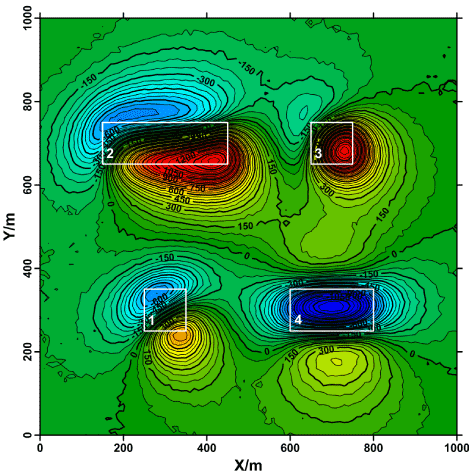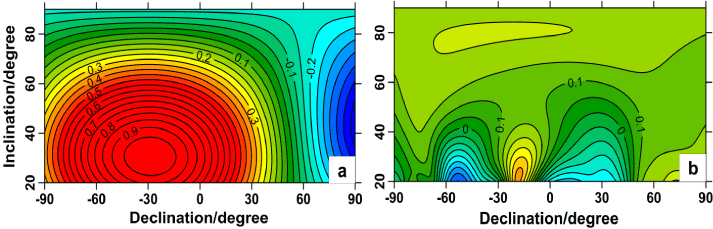Figure 1 Figure 2 Figure 3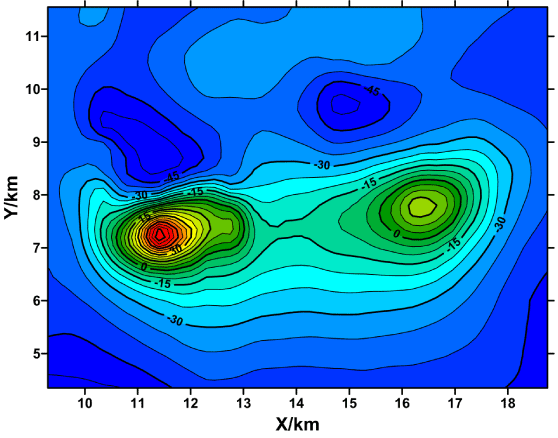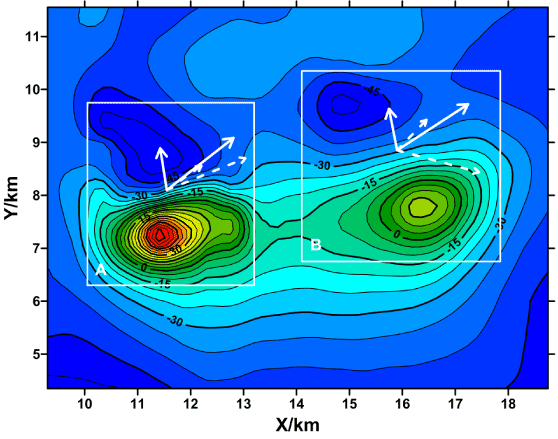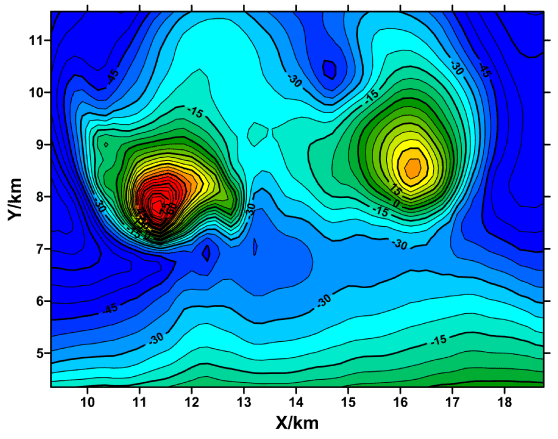Figure 4 Figure 5 Figure 6
Select your language of interest to view the total content in your interested language

### Article Usage

• Total views: 12437
• [From(publication date):
December-2013 - Jan 21, 2020]
• Breakdown by view type
• HTML page views : 8587Can't read the image? click here to refresh